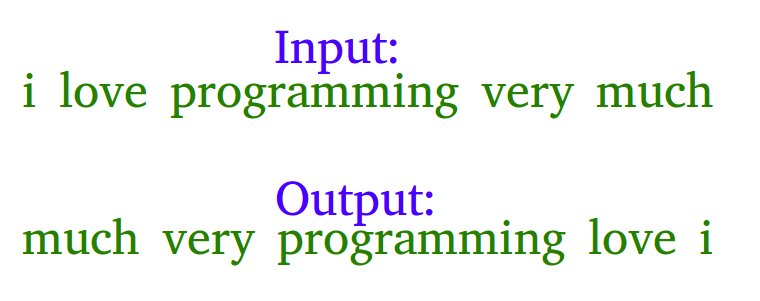# Python Program To Reverse Words In A Given String

• Last Updated : 15 Dec, 2021

Example: Let the input string be “i like this program very much”. The function should change the string to “much very program this like i”Examples

Input: s = “geeks quiz practice code”
Output: s = “code practice quiz geeks”

Input: s = “getting good at coding needs a lot of practice”
Output: s = “practice of lot a needs coding at good getting”

Algorithm:

• Initially, reverse the individual words of the given string one by one, for the above example, after reversing individual words the string should be “i ekil siht margorp yrev hcum”.
• Reverse the whole string from start to end to get the desired output “much very program this like i” in the above example.

Below is the implementation of the above approach:

## Python3

 `# Python3 program to reverse a string`` ` `# Function to reverse each word in ``# the string``def` `reverse_word(s, start, end):``    ``while` `start < end:``        ``s[start], s[end] ``=` `s[end], s[start]``        ``start ``=` `start ``+` `1``        ``end ``-``=` `1`` ` `s ``=` `"i like this program very much"`` ` `# Convert string to list to use it as ``# a char array``s ``=` `list``(s)``start ``=` `0``while` `True``:``     ` `    ``# We use a try catch block because for``    ``# the last word the list.index() function``    ``# returns a ValueError as it cannot find ``    ``# a space in the list``    ``try``:``        ``# Find the next space``        ``end ``=` `s.index(``' '``, start)`` ` `        ``# Call reverse_word function``        ``# to reverse each word``        ``reverse_word(s, start, end ``-` `1``)`` ` `        ``#Update start variable``        ``start ``=` `end ``+` `1`` ` `    ``except` `ValueError:`` ` `        ``# Reverse the last word``        ``reverse_word(s, start, ``len``(s) ``-` `1``)``        ``break`` ` `# Reverse the entire list``s.reverse()`` ` `# Convert the list back to``# string using string.join() function``s ``=` `"".join(s)`` ` `print``(s)`` ` `# This code is contributed by Prem Nagdeo`

Output:

`much very program this like i`

Another Approach:

we can do the above task by splitting and saving the string in a reverse manner.

Below is the implementation of the above approach:

## Python3

 `# Python3 program to reverse a string``# s = input()``s ``=` `"i like this program very much"``words ``=` `s.split(``' '``)``string ``=``[]``for` `word ``in` `words:``    ``string.insert(``0``, word)`` ` `print``(``"Reversed String:"``)``print``(``" "``.join(string))`` ` `# Solution proposed bu Uttam`

Output:

```Reversed String:
much very program this like i```

Time Complexity: O(n)

Without using any extra space:
The above task can also be accomplished by splitting and directly swapping the string starting from the middle. As direct swapping is involved, less space is consumed too.

Below is the implementation of the above approach:

## Python3

 `# Python3 code to reverse a string`` ` `# Reverse the string``def` `RevString(s,l):``   ` `  ``# Check if number of words is even``  ``if` `l``%``2` `=``=` `0``:``     ` `    ``# Find the middle word``    ``j ``=` `int``(l``/``2``)``     ` `    ``# Starting from the middle ``    ``# start swapping words``    ``# at jth position and l-1-j position``    ``while``(j <``=` `l ``-` `1``):``      ``s[j], s[l ``-` `j ``-` `1``] ``=` `s[l ``-` `j ``-` `1``], s[j]``      ``j ``+``=` `1``       ` `  ``# Check if number of words is odd``  ``else``:``     ` `    ``# Find the middle word``    ``j ``=` `int``(l``/``2` `+` `1``)``     ` `    ``# Starting from the middle ``    ``# start swapping the words``    ``# at jth position and l-1-j position``    ``while``(j <``=` `l ``-` `1``):``      ``s[j], s[l ``-` `1` `-` `j] ``=` `s[l ``-` `j ``-` `1``], s[j]``      ``j ``+``=` `1``     ` `    ``# return the reversed sentence``    ``return` `s;``       ` `# Driver Code``s ``=` `'getting good at coding needs a lot of practice'``string ``=` `s.split(``' '``)``string ``=` `RevString(string,``len``(string))``print``(``" "``.join(string))`

Output:

`practice of lot a needs coding at good getting`

Please refer complete article on Reverse words in a given string for more details!

My Personal Notes arrow_drop_up